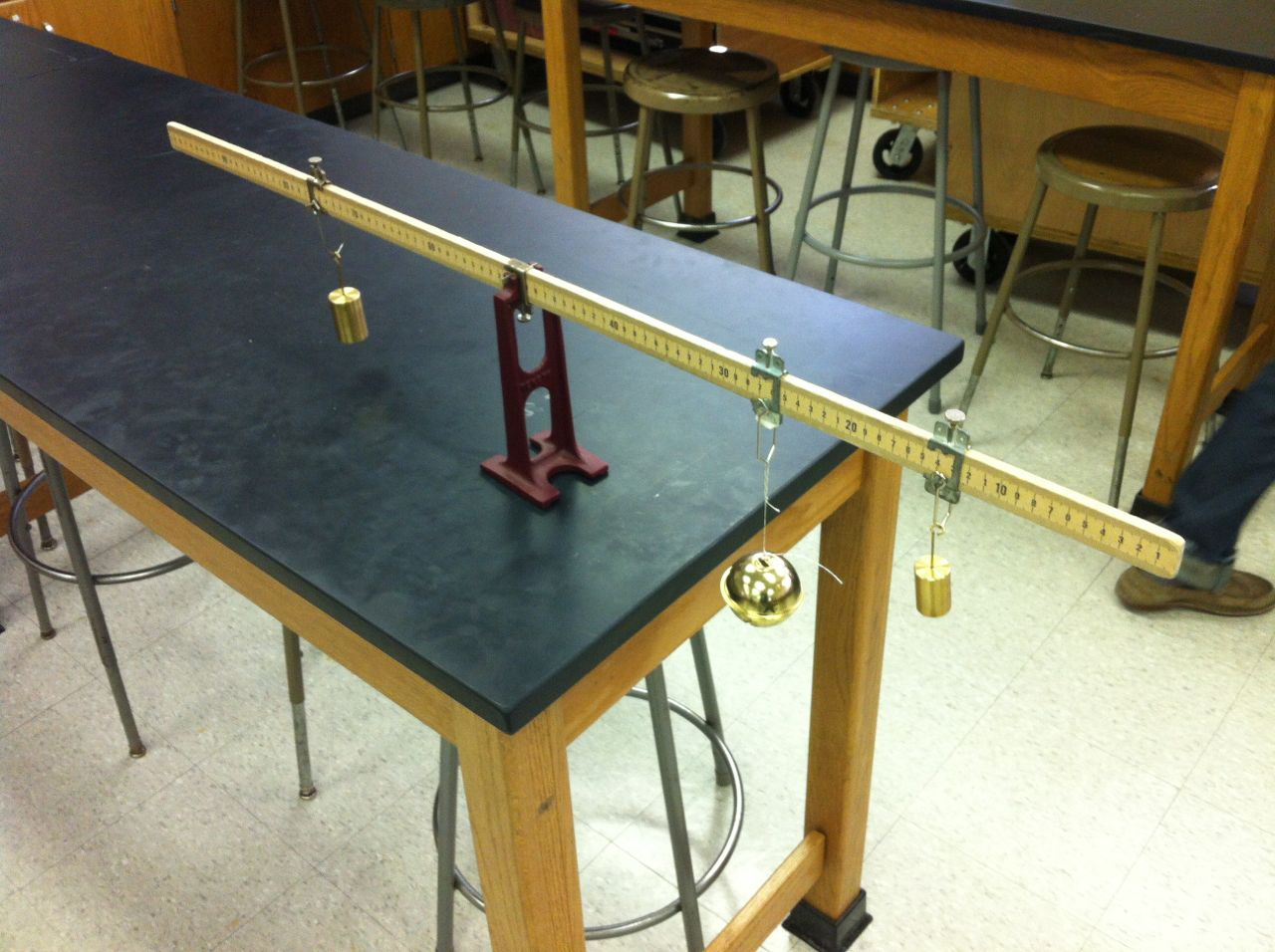# Day 110: Rotational Equilibrium (Balanced Torques)

Today students practiced analyzing a system in rotational equilibrium. We had them hang two masses on the stick and calculate where a third mass should be placed such that the meter stick would be balanced. We then had students hang an object of unknown mass and calculate its mass. This was good practice for calculating torques. It was also good practice for their upcoming lab practicum. Throughout the activity, students discovered that the system would be more stable if the bracket at the center of the meter was oriented such that the screw was facing downward. Several pairs were surprised at the inaccuracy of their prediction until they realized that they didn’t account for the mass (and torque) of the mass hangers. One common thought among students was that the mass of the mass hangers didn’t matter since all objects were hung from similar mass hangers. I let them think about this for a few seconds until they realized that each mass hanger had a different length lever arm!